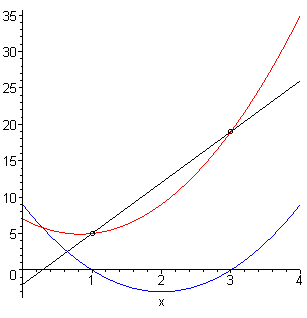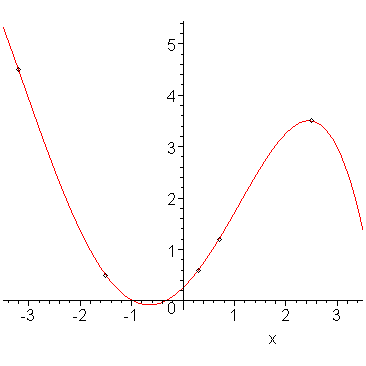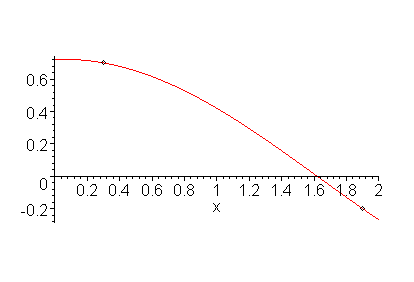# Introduction

The Vandermonde method is the most straight-forward method which may be used to find an interpolating polynomial. The substitution of the points into the desired polynomial yeilds a system of linear equations in the coefficients of the polynomial which may then be solved using Gaussian elimination or PLU decomposition.

This technique also generalizes for interpolating non-polynomial functions or points in more than two dimensions.

# Background

Useful background for this topic includes:

• Solving systems of linear equations.

# Theory

## Definition: Monomial

A monomial is a polynomial of the form xn. The degree of this monomial is n.

## Definition: Degree of a Polynomial

When a polynomial is written out in expanded form, that is, a sum of products of scalars and monomials, the degree of the polynomial is the highest degree of any monomial making up this sum.

For example, the degree of p(x) = 3.2 x7 − 4.1 x4 + 9.2 x2 + 1.2 is 7.

# The Vandermonde Method

Suppose we have 3 points (2, 5), (3, 6), (7, 4) and we want to fit a quadratic polynomial through these points. The general form of a quadratic polynomial is p(x) = c1 x2 + c2 x + c3. Thus, if we were to simply evaluate p(x) at these three points, we get three equations:

p(2) = c1 4 + c2 2 + c3 = 5
p(3) = c1 9 + c2 3 + c3 = 6
p(7) = c1 49 + c2 7 + c3 = 4

This, however, is a system of equations defined by:

where c = (c1, c2, c3)T.

Therefore, we may draw the obvious generalization that given n points (x1y1), (x2y2), ...(xnyn), we can find a polynomial of degree n − 1 which passes through those points by doing the following:

1. Writing down the general polynomial of degree n − 1,
2. Evaluating the polynomial at the points x1, ..., xn, and
3. Solving the resulting system of linear equations.

Rather than performing all of these operations, we will simply write down the problem in the form Vc = y where y is the vector of y values, c is the vector of coefficients, and V is the Vandermonde matrix.

## The Vandermonde Matrix

The Vandermonde matrix is an n × n matrix where the first row is the first point evaluated at each of the n monomials, the second row is the second point x2 evaluated at each of the n monomials, and so on.

The easiest way to create this matrix is to write the functions above the matrix and the points to the left of the matrix as is shown below. Then evaluate the functions at the corresponding points.

Thus we solve Vc = y and the entry ci is associated with the ith monomial.

# Uniqueness

We have found one polynomial of degree n − 1 which passes through n points. is it possible that there is more than one polynomial of degree n − 1 (or less) which passes through these same points? Clearly, if we have two points, there is only one straight line which passes through the two points, however, it is less obvious when there are 5 or 10 or 100 points that there exists only one unique polynomial of the desired degree which pass through those points.

## Observation

The sum of two polynomials of degree n must be of degree n or less.

## Observation

The degree of the product of two non-zero polynomials p(x) and q(x) is equal to the sum of the degrees of the two polynomials.

Example: the product of p(x) = x2 + 3x − 1 and q(x) = x7 + 3x4 − 7x + 4 is 9.

## Observation

A non-zero polynomial which has n roots must be of degree n or higher.

## Theorem

There exists a unique polynomial p(x) of degree n − 1 which passes through n points (x1, y1), ..., (xn, yn), where the xi are unique.

Proof: Suppose two polynomials of degree n − 1 pass through these n points, call them p1(x) and p2(x). Define the polynomial r(x) = p1(x) − p2(x).

This polynomial must also be of degree n − 1 or less, but it has n roots, because r(xi) = 0 for i = 1, ..., n. However, a polynomial of degree n − 1 cannot have n roots unless it is the zero polynomial.

Therefore, r(x) = 0 and therefore p1(x) = p2(x).

# Solvability

A system of linear equations is solvable if the determinant of the associated matrix is non-zero. By taking the determinant of the Vandermonde matrices of size 1×1, 2×2, and 3×3, you get the three polynomials 1, x1x2, and (x1 − x2)(x1 − x3)(x2 − x3). Extraploating this result, one could postulate that the determinant is

that is, the pairwise differences of the different x values. This can be shown through a rather painful process of mathematical induction. If two x values are equal, the determinant is zero; otherwise, the determinant must be non-zero.

# Problem 1

Find an interpolating polynomial which passes through n points (x1, y1), ..., (xn, yn).

# Assumptions

The x values are unique.

# Process

Because there are n points, the degree of the interpolating polynomial is n - 1. Thus, the interpolating polynomial is of the form:

p(x) = c1 xn - 1 + c2 xn - 2 + ⋅⋅⋅ + cn - 1 x + cn.

Next we define the n × n Vandermonde matrix V by lining up the xi's along the left of the matrix, the monomials xn - 1, ..., x, 1 along the top of the matrix, and then filling in the matrix by evaluating the point at the monomials:

NOTE: the right-hand column is 1s if and only if the last function is the constant function 1.

For example, the Vandermonde matrix for finding the polynomial which interpolates the 5 points (-3, 2), (-2, 0), (-1, 3), (0, 7), (2, 15) is:

Note that the Vandermonde matrix is independent of the y values.

The Matlab code for this is:

```x = [-3 -2 -1 0 2]';
V = [x.^4 x.^3 x.^2 x x.^0];   % or V = vander( x );
y = [2 0 3 7 15]';
c = V \ y;
```

# Problem 2

Find an interpolating function of the form

f(x) = f1(x) + f2(x) + ⋅⋅⋅ + fn - 1(x) + fn(x)

which passes through n points (x1, y1), ..., (xn, yn).

Note that the polynomial case is simply a special case where f1(x) = xn - 1, ..., fn(x) = 1.

# Assumptions

The x values are unique and the functions are linearly independent.

# Process

We define the n × n modified Vandermonde matrix V by lining up the xi's along the left of the matrix, the functions f1(x), ..., fn(x) along the top of the matrix, and then filling in the matrix by evaluating the points at the corresponding functions:

For example, the generalized Vandermonde matrix for finding the function of the form f(x) = c1 + c2sin(x) + c3cos(x) which interpolates the 3 points (4, 0.3), (5, 0.9), (6, -0.2) is:

where you should recall that we are using radians.

The Matlab code for this is:

```x = [4 5 6]';
y = [0.3 0.9 -0.2]';
V = [x.^0, sin(x), cos(x)];
c = V \ y;
```

# Error Analysis

Suppose that we have a function f(x) which is sampled n times, resulting in the points (x1y1), ..., (xnyn), where x1 < x2 < ⋅⋅⋅ < xn and yi = f(xi). We can interpolate those points using a polynomial of degree n − 1, but of interest is also the error associated with the interpolating polynomial p(x).

Thus, given a point x, the error of the interpolating polynomial p(x) is given by the formula:

where

π(x) = (xx1)(xx2)⋅⋅⋅(xxn)

and ξ ∈ [min{x1x}, max{xnx}].

Note that if the point x = xi for some i = 1, 2, ..., n, then the error is zero and that if the point x ∈ [x1xn], then the error is minimized, as ξ at this point comes from the smallest possible interval.

As an example, suppose you are interpolating the quadratic function 3x2 − 5x + 7 at the points (1,5) and (3,19). This is the linear function 7x − 2. To find the error, because we are interpolating n = 2 points, it follows that we must take the 2nd derivative which in this case is exactly 6.

Therefore, the error term is 6(x - 1)(x - 3)/2! = 3(x - 1)(x - 3). The plot in Figure 1 shows the function, the approximation, and the error. In this case, the error is exact, however, this example does try to show that the formula makes sense for a straight-forward case.Figure 1. A function, its approximation, and the error.

It is interesting to note that in this case, because the 2nd derivative is a constant, that (3x2 − 5x + 7) − (7x − 2) = 3(x − 1)(x − 3).

## Definition: extrapolation

Extrapolation is using a set of points (x1, y1), ..., (xn, yn) to estimate a point outside the interval [x1xn].

# Example 1

Find the polynomial which interpolates the points (2, 2), (3, 1), (5, 2).

Because there are three points, the interpolating polynomial is a quadratic of the form p(x) = c1x2 + c2x + c3. Thus, we define the Vandermonde matrix

and solve the system Vc = y where y = (2, 1, 2)T. This gives the result c = (0.5, -3.5, 7)T, and therefore the interpolating polynomial is p(x) = 0.5 x2 - 3.5 x + 7.

# Example 2

Find the polynomial which interpolates the points (-2, -39), (0, 3), (1, 6), (3, 36).

Because there are four points, the interpolating polynomial is a cubic of the form p(x) = c1x3 + c2x2 + c3x + c4. Thus, we define the Vandermonde matrix

and solve the system Vc = y where y = (-39, 3, 6, 36)T. This gives the result c = (2, -4, 5, 3)T, and therefore the interpolating polynomial is p(x) = 2 x3 - 4 x2 + 5 x + 3.

# Example 3

The voltages associated with various offsets of a device were sampled as (2, 4) and (3, 12). The device is known to have zero voltage when the offset is zero. Find an interpolating polynomial.

We could approach this question in one of two ways: first, we could interpolate the points (0, 0), (2, 4), (3, 12), however, we could also interpolate a polynomial of the form p(x) = c1x2 + c2x. Thus, we define the Vandermonde matrix

and solve the system Vc = y where y = (4, 12)T. This gives the result c = (2, -2)T, and therefore the interpolating polynomial is p(x) = 2 x2 - 2 x.

# Example 4

Use Matlab to find the polynomial which interpolates the points (-3.2, 4.5), (-1.5, 0.5), (0.3, 0.6), (0.7, 1.2), (2.5, 3.5). Plot the polynomial and the points and comment.

We may use the vander function as follows:

```>> x = [-3.2, -1.5, 0.3, 0.7, 2.5]';
>> y = [ 4.5,  0.5, 0.6, 1.2, 3.5]';
>> V = vander( x )
V =

1.0e+02  *

1.04858  -0.32768   0.10240  -0.03200   0.01000
0.05063  -0.03375   0.02250  -0.01500   0.01000
0.00008   0.00027   0.00090   0.00300   0.01000
0.00240   0.00343   0.00490   0.00700   0.01000
0.39062   0.15625   0.06250   0.02500   0.01000

>> V \ y
ans =

-0.03181
-0.12578
0.64266
0.97516
0.25327
```

Therefore, the interpolating polynomial is of the form p(x) = -0.03181 x4 - 0.12578 x3 + 0.64266 x2 + 0.97516 x + 0.25327. Figure 1 shows the points and the interpolating polynomial.Figure 1. The quintic polynomial passing through the points specified in Example 4.

Note that the points look more quadratic in nature than quintic? In the topic on least squares, we will see how we can fit a quadratic function to this data.

# Example 5

Find the interpolating function of the form f(x) = c1 sin(x) + c2 cos(x) which passes through the points (0.3, 0.7) and (1.9, -0.2).

The generalized Vandermonde matrix may be found using Matlab:

```x = [0.3  1.9]';
y = [0.7 -0.2]';
V = [sin(x) cos(x)]
V =
0.29552   0.95534
0.94630  -0.32329

>> c = V \ y
c =
0.03525
0.72182
```

Thus, the interpolating function is f(x) = 0.03525 sin(x) + 0.72182 cos(x) and this function and the points are shown in Figure 2.Figure 2. The sinusoidal function passing thought two points.

# Question 1

Find the linear polynomial which interpolates the points (2, 3) and (5, 7).

Answer: p(x) = 4/3 x + 1/3

# Question 2

Find the cubic polynomial which interpolates the points (-2, 21), (0, 1), (1, 0), (3, -74).

Answer: p(x) = -3 x3 + 2 x + 1

# Question 3

Find the cubic polynomial which interpolates the points (1, 5), (2, 7), (4, 11), (6, 15).

Answer: p(x) = 2 x + 3

# Question 4

Use Matlab to find the polynomial which interpolates the points

(1.3, 0.51), (0.57, 0.98), (-0.33, 1.2), (-1.2, 14), (2.1, -0.35), (0.36, 0.52)

Answer: p(x) = 0.82168 x5 - 0.99000 x4 - 4.54270 x3 + 6.48945 x2 - 0.64121 x + 0.13341

# Question 5

Must the x values be ordered from smallest to largest for the Vandermonde method to work? (Try this in Matlab with one of the examples.)

# Applications to Engineering

The Vandermonde technique, while very straight-forward for polynomials, can also be easily applied to fitting any type of functions, so long as they satisfy some basic conditions. For example, it may be useful to interpolate exponential or sinusoidal functions.

# Matlab

The vander routine automatically generates a Vandermonde matrix:

```>> x = [1.2 1.5 1.9 2.5 2.6]'
>> y = [0.5 0.3 0.5 0.2 0.7]'
>> V = vander( x )
>> c = V \ y
```

though this may be done manually, as well:

```>> V = [x.^4 x.^3 x.^2 x x.^0]       % element-wise exponentiation
>> c = V \ y
```

This can be generalized: suppose you wanted to interpolate the function f(x) = c1 e-x + c2:

```>> V = [exp(-x) x.^0]
>> c = V \ y
```

# Maple

Finding an interpolating polynomial with Maple may be done with the PolynomialInterpolation routine:

```CurveFitting[PolynomialInterpolation]( [[0.3, 1.5], [0.4, 1.7], [0.6, 2.5]], x );
plot( %, x = 0..1 );
```

For more help on either of this routine or on the CurveFitting package, enter:

```?CurveFitting
?CurveFitting,PolynomialInterpolation
```